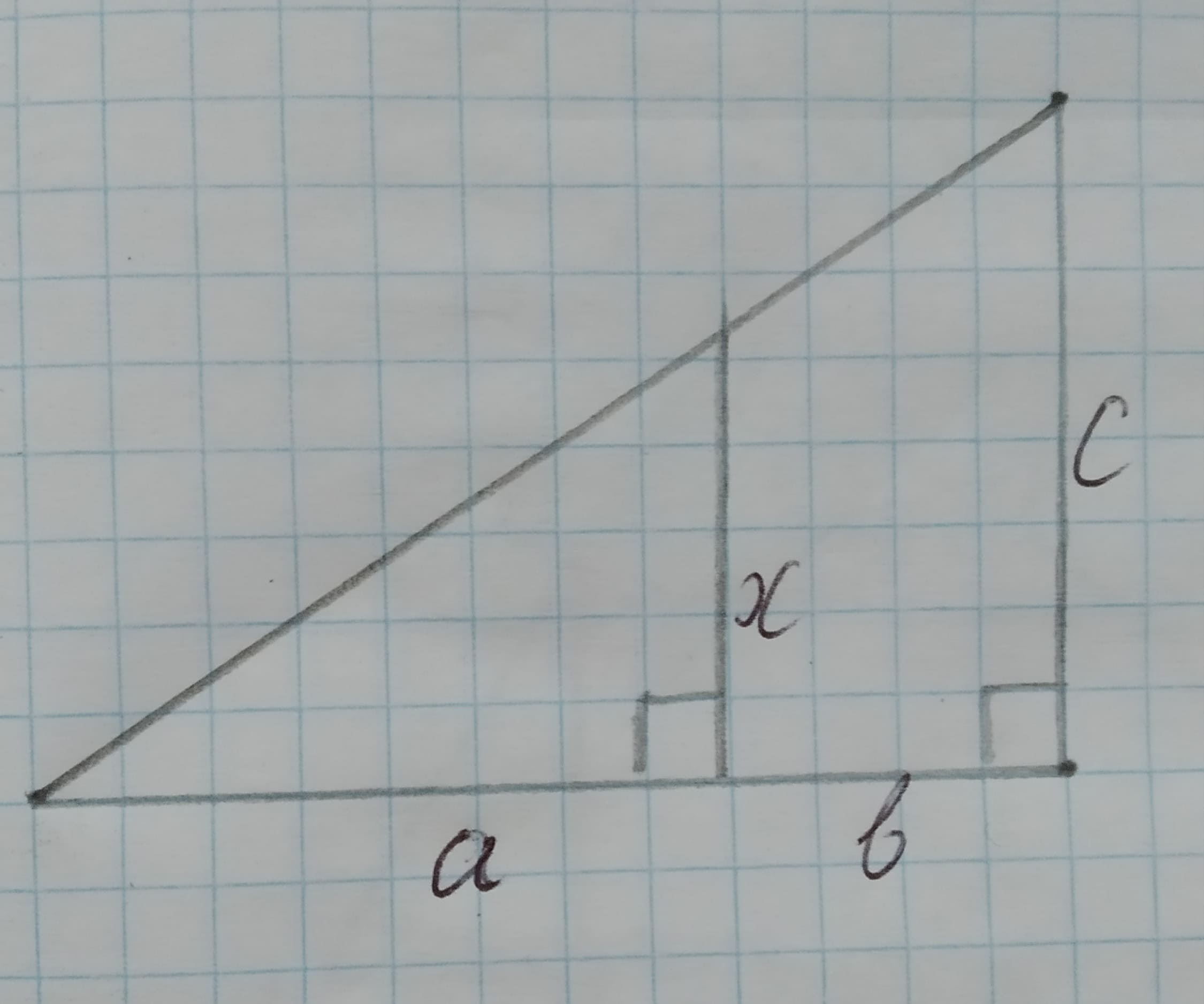# Using Similarity Express x in terms of a, b, and c.Given:The given pair of triangles is similar.The given pair of triangle is,12210202671.jpgDaniaal Sanchez 2020-10-28 Answered

Using Similarity Express x in terms of a, b, and c.
Given:
The given pair of triangles is similar.
The given pair of triangle is,You can still ask an expert for help

• Questions are typically answered in as fast as 30 minutes

Solve your problem for the price of one coffee

• Math expert for every subject
• Pay only if we can solve itsweererlirumeX
Approach:
Two triangles are similar if their vertices can be matched up so that corresponding angles are congruent. In this case corresponding sides are proportional.
Calculation:
By similarity,
The lengths of the corresponding sides in the triangles are proportional.
$x=a=\frac{c}{a+b}$
$x=a\left(\frac{c}{a+b}\right)$
$x=\frac{ac}{a+b}$
Therefore, the required expression is $x=\frac{ac}{a+b}$.
Conclusion:
Hence, the required expression is $x=\frac{ac}{a+b}$.